# Black body

﻿
Black body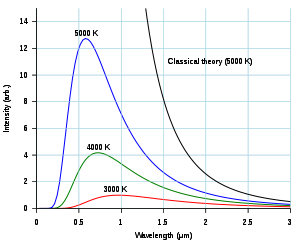As the temperature decreases, the peak of the blackbody radiation curve moves to lower intensities and longer wavelengths. The blackbody radiation graph is also compared with the classical model of Rayleigh and Jeans.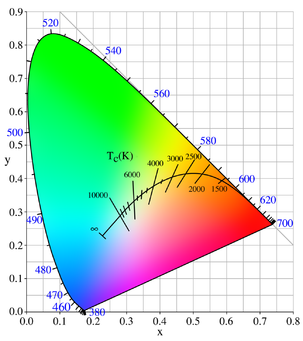The color (chromaticity) of blackbody radiation depends on the temperature of the black body; the locus of such colors, shown here in CIE 1931 x,y space, is known as the Planckian locus.

A black body is an idealized physical body that absorbs all incident electromagnetic radiation. Because of this perfect absorptivity at all wavelengths, a black body is also the best possible emitter of thermal radiation, which it radiates incandescently in a characteristic, continuous spectrum that depends on the body's temperature. At Earth-ambient temperatures this emission is in the infrared region of the electromagnetic spectrum and is not visible. The object appears black, since it does not reflect or emit any visible light.

The thermal radiation from a black body is energy converted electrodynamically from the body's pool of internal thermal energy at any temperature greater than absolute zero. It is called blackbody radiation and has a frequency distribution with a characteristic frequency of maximum radiative power that shifts to higher frequencies with increasing temperature. As the temperature increases past a few hundred degrees Celsius, black bodies start to emit visible wavelengths, appearing red, orange, yellow, white, and blue with increasing temperature. When an object is visually white, it is emitting a substantial fraction as ultraviolet radiation.

Blackbody emission provides insight into the thermodynamic equilibrium state of cavity radiation. If each Fourier mode of the absolutely stable equilibrium radiation in a cavity with perfectly reflective walls were considered as a degree of freedom, and if all those degrees of freedom could freely exchange energy, then, according to the equipartition theorem in classical physics, each degree of freedom would have one and the same quantity of energy. This approach led to the paradox known as the ultraviolet catastrophe, that there would be an infinite amount of energy in any continuous field. The study of the laws of black bodies helped to establish the foundations of quantum mechanics.

The term black body was introduced by Gustav Kirchhoff in 1860. When used as a compound adjective, the term is typically written as one word in English, e.g. in blackbody radiation, but sometimes also hyphenated, as in black-body radiation.

## Explanation

All matter emits electromagnetic radiation when it has a temperature above absolute zero. The radiation represents a conversion of a body's thermal energy into electromagnetic energy, and is therefore called thermal radiation. It is a spontaneous process of radiative distribution of entropy.

Conversely all matter absorbs electromagnetic radiation to some degree. An object that absorbs all radiation falling on it, at all wavelengths, is called a black body. When a black body is at a uniform temperature, its emission has a characteristic frequency distribution that depends on the temperature. Its emission is called blackbody radiation.

The concept of the black body is an idealization, as perfect black bodies do not exist in nature. Graphite and lamp black, with emissivities greater than 0.95, however, are good approximations to a black material. Experimentally, blackbody radiation may be established best as the ultimately stable steady state equilibrium radiation in a cavity in a rigid body, at a uniform temperature, that is entirely opaque and is only partly reflective. A closed box of graphite walls at a constant temperature with a small hole on one side produces a good approximation to ideal blackbody radiation emanating from the opening.

Blackbody radiation has the unique absolutely stable distribution of radiative intensity that can persist in thermodynamic equilibrium in a cavity.

Blackbody radiation becomes a visible glow of light if the temperature of the object is high enough. The Draper point is the temperature at which all solids glow a dim red, about 798 K. At 1000 K, the opening in the oven looks red; at 6000 K, it looks white. No matter how the oven is constructed, or of what material, as long as it is built such that almost all light entering is absorbed, it will be a good approximation to a blackbody, so the spectrum, and therefore color, of the light that comes out will be a function of the cavity temperature alone. A graph of the amount of energy inside the oven per unit volume and per unit frequency interval plotted versus frequency, is called the blackbody curve. Different curves are obtained by varying the temperature.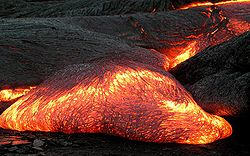The temperature of a Pāhoehoe lava flow can be estimated by observing its color. The result agrees well with measured temperatures of lava flows at about 1000 to 1200 °C.

Two things that are at the same temperature stay in equilibrium, so a body at temperature T surrounded by a cloud of light at temperature T on average will emit as much light into the cloud as it absorbs, following Prevost's exchange principle, which refers to radiative equilibrium. The principle of detailed balance says that there are no strange correlations between the process of emission and absorption: the process of emission is not affected by the absorption, but only by the thermal state of the emitting body. This means that the total light emitted by a body at temperature T, black or not, is always equal to the total light that the body would absorb were it to be surrounded by light at temperature T.

When the body is black, the absorption is obvious: the amount of light absorbed is all the light that hits the surface. For a black body much bigger than the wavelength, the light energy absorbed at any wavelength λ per unit time is strictly proportional to the blackbody curve. This means that the blackbody curve is the amount of light energy emitted by a black body, which justifies the name. This is the condition for the applicability of Kirchhoff's law of thermal radiation: the blackbody curve is characteristic of thermal light, which depends only on the temperature of the walls of the cavity, provided that the walls of the cavity are completely opaque and are not very reflective, and that the cavity is in thermodynamic equilibrium. When the black body is small, so that its size is comparable to the wavelength of light, the absorption is modified, because a small object is not an efficient absorber of light of long wavelength, but the principle of strict equality of emission and absorption is always upheld in a condition of thermodynamic equilibrium.

In the laboratory, blackbody radiation is approximated by the radiation from a small hole in a large cavity, a hohlraum, in an entirely opaque body that is only partly reflective, that is maintained at a constant temperature. (This technique leads to the alternative term cavity radiation.) Any light entering the hole would have to reflect off the walls of the cavity multiple times before it escaped, in which process it is nearly certain to be absorbed. Absorption occurs regardless of the wavelength of the radiation entering (as long as it is small compared to the hole). The hole, then, is a close approximation of a theoretical black body and, if the cavity is heated, the spectrum of the hole's radiation (i.e., the amount of light emitted from the hole at each wavelength) will be continuous, and will depend only on the opacity and partial reflectivity of the walls, but not on the particular material of which they are built nor on the material in the cavity (compare with emission spectrum).

Calculating the blackbody curve was a major challenge in theoretical physics during the late nineteenth century. The problem was solved in 1901 by Max Planck in the formalism now known as Planck's law of blackbody radiation. By making changes to Wien's radiation law (not to be confused with Wien's displacement law) consistent with thermodynamics and electromagnetism, he found a mathematical expression fitting the experimental data satisfactorily. Planck had to assume that the energy of the oscillators in the cavity was quantized, i.e., it existed in integer multiples of some quantity. Einstein built on this idea and proposed the quantization of electromagnetic radiation itself in 1905 to explain the photoelectric effect. These theoretical advances eventually resulted in the superseding of classical electromagnetism by quantum electrodynamics. These quanta were called photons and the blackbody cavity was thought of as containing a gas of photons. In addition, it led to the development of quantum probability distributions, called Fermi–Dirac statistics and Bose–Einstein statistics, each applicable to a different class of particles, fermions and bosons.

The wavelength at which the radiation is strongest is given by Wien's displacement law, and the overall power emitted per unit area is given by the Stefan–Boltzmann law. So, as temperature increases, the glow color changes from red to yellow to white to blue. Even as the peak wavelength moves into the ultra-violet, enough radiation continues to be emitted in the blue wavelengths that the body will continue to appear blue. It will never become invisible—indeed, the radiation of visible light increases monotonically with temperature.

The radiance or observed intensity is not a function of direction. Therefore a black body is a perfect Lambertian radiator.

Real objects never behave as full-ideal black bodies, and instead the emitted radiation at a given frequency is a fraction of what the ideal emission would be. The emissivity of a material specifies how well a real body radiates energy as compared with a black body. This emissivity depends on factors such as temperature, emission angle, and wavelength. However, it is typical in engineering to assume that a surface's spectral emissivity and absorptivity do not depend on wavelength, so that the emissivity is a constant. This is known as the gray body assumption.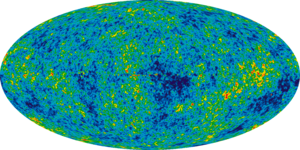The distribution of the cosmic microwave background radiation across the universe as measured by the Wilkinson Microwave Anisotropy Probe. It is the most precisely known thermal emission spectrum and corresponds to a temperature of 2.725 K and an emission peak frequency of 160.2 GHz.

With non-black surfaces, the deviations from ideal blackbody behavior are determined by both the surface structure, such as roughness or granularity, and the chemical composition. On a "per wavelength" basis, real objects in states of local thermodynamic equilibrium still follow Kirchhoff's Law: emissivity equals absorptivity, so that an object that does not absorb all incident light will also emit less radiation than an ideal black body; the incomplete absorption can be due to some of the incident light being transmitted through the body or to some of it being reflected at the surface of the body.

In astronomy, objects such as stars are frequently regarded as black bodies, though this is often a poor approximation. An almost perfect blackbody spectrum is exhibited by the cosmic microwave background radiation. Hawking radiation is the hypothetical blackbody radiation emitted by black holes.

A black body radiates energy at all frequencies, but its intensity rapidly tends to zero at high frequencies (short wavelengths). For example, a black body at room temperature (300 K) with one square meter of surface area will emit a photon in the visible range (390–750 nm) at an average rate of one photon every 41 seconds, meaning that for most practical purposes, such a black body does not emit in the visible range.

## Blackbody simulators

A black body is an idealized object, and by definition has an emissivity of e = 1.0. In practice, common applications define all sources of infrared radiation as a black body when the object approaches that emissivity and is greater than approximately 0.99. A source with lower emissivity is often referred to as a gray body.

An example of a nearly perfect black body is super black, produced from a nickel-phosphorus alloy. In 2009, a team of Japanese scientists created a material even closer to an ideal black body, based on vertically aligned single-walled carbon nanotubes. This absorbs between 98% and 99% of the incoming light in the spectral range from the ultra-violet to the far-infrared regions.

## Equations governing black bodies

### Planck's law of blackbody radiation

Planck's law states that$I(\nu,T) =\frac{ 2 h\nu^{3}}{c^2}\frac{1}{ e^{\frac{h\nu}{kT}}-1}.$

where

I(ν,T) is the energy per unit time (or the power) radiated per unit area of emitting surface in the normal direction per unit solid angle per unit frequency by a black body at temperature T;
h is the Planck constant;
c is the speed of light in a vacuum;
k is the Boltzmann constant;
ν is the frequency of the electromagnetic radiation; and
T is the temperature of the body in kelvins.

### Wien's displacement law

Wien's displacement law shows how the spectrum of black body radiation at any temperature is related to the spectrum at any other temperature. If we know the shape of the spectrum at one temperature, we can calculate the shape at any other temperature.

A consequence of Wien's displacement law is that the wavelength at which the intensity of the radiation produced by a black body is at a maximum, λmax , it is a function only of the temperature$\lambda_\max = \frac{b}{T}$

where the constant, b, known as Wien's displacement constant, is equal to 2.8977721(26)×10−3 K m.

Note that the peak intensity can be expressed in terms of intensity per unit wavelength or in terms of intensity per unit frequency. The expression for the peak wavelength given above refers to the intensity per unit wavelength; meanwhile the Planck's Law section above was in terms of intensity per unit frequency. The frequency at which the power per unit frequency is maximised is given by$\nu_\max = T \times 103.5\ \mathrm{GHz}\mathrm{ K}^{-1}$.

### Stefan–Boltzmann law

The Stefan–Boltzmann law states that the power emitted per unit area of the surface of a black body is directly proportional to the fourth power of its absolute temperature:$j^{\star} = \sigma T^4,$

where j*is the total power radiated per unit area, T is the absolute temperature and σ = 5.67×10−8 W m−2 K−4 is the Stefan–Boltzmann constant.

## Human body emission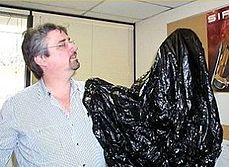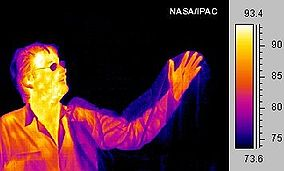Much of a person's energy is radiated away in the form of infrared light. Some materials are transparent in the infrared, while opaque to visible light, as is the plastic bag in this infrared image (bottom). Other materials are transparent to visible light, while opaque or reflective in the infrared, noticeable by darkness of the man's glasses.

As all matter, the human body radiates some of a person's energy away as infrared light.

The net power radiated is the difference between the power emitted and the power absorbed:$P_\mathrm{net}=P_\mathrm{emit}-P_\mathrm{absorb}. \,$

Applying the Stefan–Boltzmann law,$P_{\rm net}=A\sigma \varepsilon \left( T^4 - T_0^4 \right).$

The total surface area of an adult is about 2 m2, and the mid- and far-infrared emissivity of skin and most clothing is near unity, as it is for most nonmetallic surfaces. Skin temperature is about 33 °C, but clothing reduces the surface temperature to about 28 °C when the ambient temperature is 20 °C. Hence, the net radiative heat loss is about$P_{\rm net} = 100 \ \mathrm{W}.$

The total energy radiated in one day is about 9 MJ (megajoules), or 2000 kcal (food calories). Basal metabolic rate for a 40-year-old male is about 35 kcal/(m2·h), which is equivalent to 1700 kcal per day assuming the same 2 m2 area. However, the mean metabolic rate of sedentary adults is about 50% to 70% greater than their basal rate.

There are other important thermal loss mechanisms, including convection and evaporation. Conduction is negligible – the Nusselt number is much greater than unity. Evaporation via perspiration is only required if radiation and convection are insufficient to maintain a steady state temperature (but evaporation from the lungs occurs regardless). Free convection rates are comparable, albeit somewhat lower, than radiative rates. Thus, radiation accounts for about two-thirds of thermal energy loss in cool, still air. Given the approximate nature of many of the assumptions, this can only be taken as a crude estimate. Ambient air motion, causing forced convection, or evaporation reduces the relative importance of radiation as a thermal loss mechanism.

Application of Wien's Law to human body emission results in a peak wavelength of$\lambda_{\rm peak} = \frac{2.898\times 10^6 \ \mathrm{K} \cdot \mathrm{nm}}{305 \ \mathrm{K}} = 9500 \ \mathrm{nm}.$

For this reason, thermal imaging devices for human subjects are most sensitive in the 7000–14000 nanometer range.

## Temperature relation between a planet and its star

The blackbody law may be used to estimate the temperature of a planet orbiting the Sun.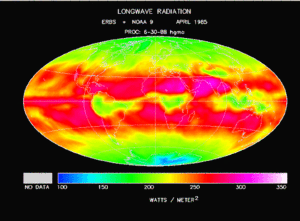Earth's longwave thermal radiation intensity, from clouds, atmosphere and ground

The temperature of a planet depends on several factors:

This example is concerned with the balance of incident and emitted radiation, which is the most important impact for the inner planets in the Solar System.

The Stefan–Boltzmann law gives the total power (energy/second) the Sun is emitting:The Earth only has an absorbing area equal to a two dimensional disk, rather than the surface of a sphere.$P_{\rm S\ emt} = 4 \pi R_{\rm S}^2 \sigma T_{\rm S}^4 \qquad \qquad (1)$

where$\sigma \,$ is the Stefan–Boltzmann constant,$T_{\rm S} \,$ is the effective temperature of the Sun, and$R_{\rm S} \,$ is the radius of the Sun.

The Sun emits that power equally in all directions. Because of this, the planet is hit with only a tiny fraction of it. The power from the Sun that strikes the planet (at the top of the atmosphere) is:$P_{\rm SE} = P_{\rm S\ emt} \left( \frac{\pi R_{\rm E}^2}{4 \pi D^2} \right) \qquad \qquad (2)$

where$R_{\rm E} \,$ is the radius of the planet and$D \,$ is the astronomical unit, the distance between the Sun and the planet.

Because of its high temperature, the sun emits to a large extent in the ultraviolet and visible (UV-Vis) frequency range. In this frequency range, the planet reflects a fraction α of this energy where α is the albedo or reflectance of the planet in the UV-Vis range. In other words, the planet absorbs a fraction 1 − α of the sun's light, and reflects the rest. The power absorbed by the planet and its atmosphere is then:$P_{\rm abs} = (1-\alpha)\,P_{\rm SE} \qquad \qquad (3)$

Even though the planet only absorbs as a circular area πR2, it emits equally in all directions as a sphere. If the planet were a perfect black body, it would emit according to the Stefan–Boltzmann law$P_{\rm emt\,bb} = 4 \pi R_{\rm E}^2 \sigma T_{\rm E}^4 \qquad \qquad (4)$

where TE is the temperature of the planet. This temperature, calculated for the case of the planet acting as a black body by setting$P_{\rm abs} = P_{\rm emt\,bb}$, is known as the effective temperature. The actual temperature of the planet will likely be different, depending on its surface and atmospheric properties. Ignoring the atmosphere and greenhouse effect, the planet, since it is at a much lower temperature than the sun, emits mostly in the infrared (IR) portion of the spectrum. In this frequency range, it emits$\overline{\epsilon}$ of the radiation that a black body would emit where$\overline{\epsilon}$ is the average emissivity in the IR range. The power emitted by the planet is then:$P_{\rm emt} = \overline{\epsilon}\,P_{\rm emt\,bb} \qquad \qquad (5)$

For a body in radiative exchange equilibrium with its surroundings, the rate at which it emits radiant energy is equal to the rate at which it absorbs it:$P_{\rm abs}=P_{\rm emt} \qquad \qquad (6)$

Substituting the expressions for solar and planet power in equations 1–6 and simplifying yields the estimated temperature of the planet, ignoring greenhouse effect, TP:$T_P = T_S\sqrt{\frac{R_S\sqrt{\frac{1-\alpha}{\overline{\varepsilon}}}}{2D}}$

In other words, given the assumptions made, the temperature of a planet depends only on the surface temperature of the Sun, the radius of the Sun, the distance between the planet and the Sun, the albedo and the IR emissivity of the planet.

### Temperature of Earth

Substituting the measured values for the Sun and Earth yields:$T_{\rm S} = 5778 \ \mathrm{K},$$R_{\rm S} = 6.96 \times 10^8 \ \mathrm{m},$$D = 1.496 \times 10^{11} \ \mathrm{m},$$\alpha = 0.306 \$

With the average emissivity set to unity, the effective temperature of the Earth is:$T_{\rm E} = 254.356\ \mathrm{K}$

or −18.8 °C.

This is the temperature of the Earth if it radiated as a perfect black body in the infrared, ignoring greenhouse effects (which can raise the surface temperature of a body above what it would be if it were a perfect black body in all spectrums, with an albedo of zero), and assuming an unchanging albedo. The Earth in fact radiates not quite as a perfect black body in the infrared which will raise the estimated temperature a few degrees above the effective temperature. If we wish to estimate what the temperature of the Earth would be if it had no atmosphere, then we could take the albedo and emissivity of the moon as a good estimate. The albedo and emissivity of the moon are about 0.1054 and 0.95 respectively, yielding an estimated temperature of about 1.36 °C.

Estimates of the Earth's average albedo vary in the range 0.3–0.4, resulting in different estimated effective temperatures. Estimates are often based on the solar constant (total insolation power density) rather than the temperature, size, and distance of the sun. For example, using 0.4 for albedo, and an insolation of 1400 W m−2, one obtains an effective temperature of about 245 K. Similarly using albedo 0.3 and solar constant of 1372 W m−2, one obtains an effective temperature of 255 K.

## Doppler effect for a moving black body

The relativistic Doppler effect causes a shift in the frequency f of light originating from a source that is moving in relation to the observer, so that the wave is observed to have frequency f':$f' = f \frac{1 - \frac{v}{c} \cos \theta}{\sqrt{1-v^2/c^2}},$

where v is the velocity of the source in the observer's rest frame, θ is the angle between the velocity vector and the observer-source direction measured in the reference frame of the source, and c is the speed of light. This can be simplified for the special cases of objects moving directly towards (θ = π) or away (θ = 0) from the observer, and for speeds much less than c.

Through Planck's law the temperature spectrum of a black body is proportionally related to the frequency of light and one may substitute the temperature (T) for the frequency in this equation.

For the case of a source moving directly towards or away from the observer, this reduces to$T' = T \sqrt{\frac{c-v}{c+v}}.$

Here v > 0 indicates a receding source, and v < 0 indicates an approaching source.

This is an important effect in astronomy, where the velocities of stars and galaxies can reach significant fractions of c. An example is found in the cosmic microwave background radiation, which exhibits a dipole anisotropy from the Earth's motion relative to this black body radiation field.

## History

### Balfour Stewart

In 1858, Balfour Stewart described his experiments on the thermal radiative emissive and absorptive powers of polished plates of various substances, compared with the powers of lamp-black surfaces, at the same temperature. Stewart chose lamp-black surfaces as his reference because of various previous experimental findings, especially those of Pierre Prevost and of John Leslie. He wrote "Lamp-black, which absorbs all the rays that fall upon it, and therefore possesses the greatest possible absorbing power, will possess also the greatest possible radiating power." More an experimenter than a logician, Stewart failed to point out that his statement presupposed an abstract general principle, that there exist either ideally in theory or really in nature bodies or surfaces that respectively have one and the same unique universal greatest possible absorbing power, likewise for radiating power, for every wavelength and equilibrium temperature.

Stewart measured radiated power with a thermo-pile and sensitive galvanometer read with a microscope. He was concerned with selective thermal radiation, which he investigated with plates of substances that radiated and absorbed selectively for different qualities of radiation rather than maximally for all qualities of radiation. He discussed the experiments in terms of rays which could be reflected and refracted, and which obeyed the Stokes-Helmholtz reciprocity principle (though he did not use an eponym for it). He did not in this paper mention that the qualities of the rays might be described by their wavelengths, nor did he use spectrally resolving apparatus such as prisms or diffraction gratings. His work was quantitative within these constraints. He made his measurements in a room temperature environment, and quickly so as to catch his bodies in a condition near the thermal equilibrium in which they had been prepared by heating to equilibrium with boiling water. His measurements confirmed that substances that emit and absorb selectively respect the principle of selective equality of emission and absorption at thermal equilibrium.

Stewart offered a theoretical proof that this should be the case separately for every selected quality of thermal radiation, but his mathematics was not rigorously valid. He made no mention of thermodynamics in this paper, though he did refer to conservation of vis viva. He proposed that his measurements implied that radiation was both absorbed and emitted by particles of matter throughout depths of the media in which it propagated. He applied the Helmholtz reciprocity principle to account for the material interface processes as distinct from the processes in the interior material. He did not postulate unrealizable perfectly black surfaces. He concluded that his experiments showed that in a cavity in thermal equilibrium, the heat radiated from any part of the interior bounding surface, no matter of what material it might be composed, was the same as would have been emitted from a surface of the same shape and position that would have been composed of lamp-black. He did not state explicitly that the lamp-black-coated bodies that he used as reference must have had a unique common spectral emittance function that depended on temperature in a unique way.

### Gustav Kirchhoff

In 1859, not knowing of Stewart's work, Gustav Robert Kirchhoff reported the coincidence of the wavelengths of spectrally resolved lines of absorption and of emission of visible light. In a second paper in 1859, Kirchhoff announced a general principle or law for which he offered a theoretical and mathematical proof, though he did not offer quantitative measurements of radiation powers. His theoretical proof was and still is considered by some writers to be invalid. His principle, however, has endured: it was that for heat rays of the same wavelength, in equilibrium at at a given temperature, the ratio of emitting power to absorption ratio has one and the same common value for all bodies that emit and absorb at that wavelength. In a notation different from Kirchhoff's, the emitting power E was a dimensioned quantity. The absorption ratio a was dimensionless, the ratio of absorbed to incident radiation, although, in contrast with Balfour Stewart's paper, not referred in particular to a lamp-black surface as the source of the incident radiation. Thus the ratio E / a of emitting power to absorption ratio was a dimensioned quantity, with the dimensions of emitting power. In this paper there was no mention of black bodies. But it was observed that the dimensioned ratio E / a, with one and the same value common to all bodies that emit and absorb, was therefore a function only of wavelength and temperature.

In 1860, still not knowing of Stewart's measurements, Kirchhoff wrote that such measurements had not been made: "darüber ist bisher weder durch theoretische Betrachtungen noch durch Versuche etawas ermittelt." Again without measurements of radiative powers or other new experimental data, Kirchhoff then offered a fresh theoretical proof of the principle of the universality of the ratio E / a at thermal equilibrium. His fresh theoretical proof was and still is considered by some writers to be invalid.

But more importantly, it relied on a new theoretical postulate of "perfectly black bodies". Such black bodies showed complete absorption in their infinitely thin most superficial surface. They correspond to Balfour Stewart's bodies, with internal radiation, coated with lamp-black. They were not the more realistic perfectly black bodies later considered by Planck. Planck's black bodies radiated and absorbed only by the material in their interiors; their interfaces with contiguous media were only mathematical surfaces, capable neither of absorption nor emission, but only of reflecting and transmitting with refraction.

Kirchhoff's proof considered an arbitrary non-ideal body as well as a perfect black body. It required that the bodies be kept in thermal equilibrium. Kirchhoff considered processes at an arbitrarily specified fixed wavelength λ and at a particular fixed temperature. A non-ideal body, possibly partly transparent and partly reflective, again had a dimensioned emissive power E . It also had a dimensionless absorption ratio a . Then he was concerned with the ratio E / a, which is a power, with the same dimensions as E, because a is dimensionless.

His proof intended to show that the ratio E / a was independent of the nature of the non-ideal body, however partly transparent or partly reflective it was. His proof first argued that for wavelength λ and at temperature T, at thermal equilibrium, all perfectly black bodies have the same emission, which is here denoted Bλ (λ, T), with the dimensions of power. His proof noted that the dimensionless absorption ratio of a perfectly black body is by definition exactly 1. Then for a perfectly black body, the ratio of emissive power to absorption ratio is again just Bλ (λ, T), with the dimensions of power. Kirchhoff considered, successively, thermal equilibrium with the arbitrary non-ideal body, and with the perfectly black body, in place in his thought-experimental set-up. He argued that the flows of heat radiation must be the same in each case. Thus he argued that at thermal equilibrium the ratio E / a was equal to Bλ (λ, T), a continuous function of λ at fixed temperature T , and an increasing function of T at fixed wavelength λ, at low temperatures vanishing for visible but not for longer wavelengths, with positive values for visible wavelengths at higher temperatures, which does not depend on the nature of the arbitrary non-ideal body. (Geometrical factors, taken into account by Kirchhoff, have been ignored in the foregoing.)

Thus Kirchhoff's law of thermal radiation can be stated: "For any material at all , radiating and absorbing in thermodynamic equilibrium at any given temperature T, for every wavelength λ, the ratio of emissive power to absorptive ratio has one universal value, which is characteristic of a perfect black body, and is an emissive power which we here represent by Bλ (λ, T) ." (For our notation Bλ (λ, T) , Kirchhoff's original notation was simply e.)

Kirchhoff announced that the determination of the function Bλ (λ, T) was a problem of the highest importance, though he recognized that there would be experimental difficulties to be overcome. He supposed that like other functions that do not depend on the properties of individual bodies, it would be a simple function. That function Bλ (λ, T) has occasionally been called 'Kirchhoff's (emission, universal) function', though its precise mathematical form would not be known for another forty years, till it was discovered by Planck in 1900. The theoretical proof for Kirchhoff's universality principle was worked on and debated by various physicists over the same time, and later. Kirchhoff stated later in 1860 that his theoretical proof was better than Balfour Stewart's, and in some respects it was so. Kirchhoff's 1860 paper did not mention the second law of thermodynamics, and of course did not mention the concept of entropy which had not at that time been established.

Wikimedia Foundation. 2010.

### Look at other dictionaries:

• black body — noun a hypothetical object capable of absorbing all the electromagnetic radiation falling on it (Freq. 9) a black body maintained at a constant temperature is a full radiator at that temperature because the radiation reaching and leaving it must… …   Useful english dictionary

• black body — visiškasis spinduolis statusas T sritis Standartizacija ir metrologija apibrėžtis Kūnas, sugeriantis visą į jį patenkančią (visų bangų ilgių, krypčių ir poliškumo) spinduliuotę. Kiekvienai pasirinktai temperatūrai kiekviename bangų ilgyje jis… …   Penkiakalbis aiškinamasis metrologijos terminų žodynas

• black body — juodasis kūnas statusas T sritis fizika atitikmenys: angl. black body vok. schwarzer Körper, m rus. чёрное тело, n pranc. corps noir, m …   Fizikos terminų žodynas

• black body — /blæk ˈbɒdi/ (say blak bodee) noun Physics 1. a body which emits thermal radiation when heated, the spectral distribution of which depends only on its temperature; full radiator. 2. a theoretical body which, if it existed, would absorb all and… …   Australian English dictionary

• Black body — Абсолютно чёрное тело …   Краткий толковый словарь по полиграфии

• black body — n. object which absorbs all wavelengths of electromagnetic radiation and is also the most efficient emitter of all wavelengths (Physics) …   English contemporary dictionary

• black body — noun Physics a hypothetical perfect absorber and radiator of energy, with no reflecting power …   English new terms dictionary

• black body radiation — noun That emitted by a black body • • • Main Entry: ↑black …   Useful english dictionary

• black body temperature — noun That at which a black body would emit the same radiation as is emitted by a given radiator at a given temperature • • • Main Entry: ↑black …   Useful english dictionary

• Black-Body Theory and the Quantum Discontinuity — 1894 1912 (1987) is a book by Thomas Kuhn. In this book, Kuhn looks at the early development of quantum mechanics through the work of Max Planck. Kuhn s major thesis is that even after Planck arrived at his quantum treatment of the irreversibili …   Wikipedia